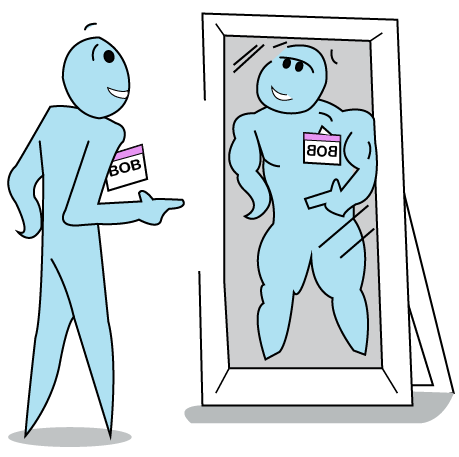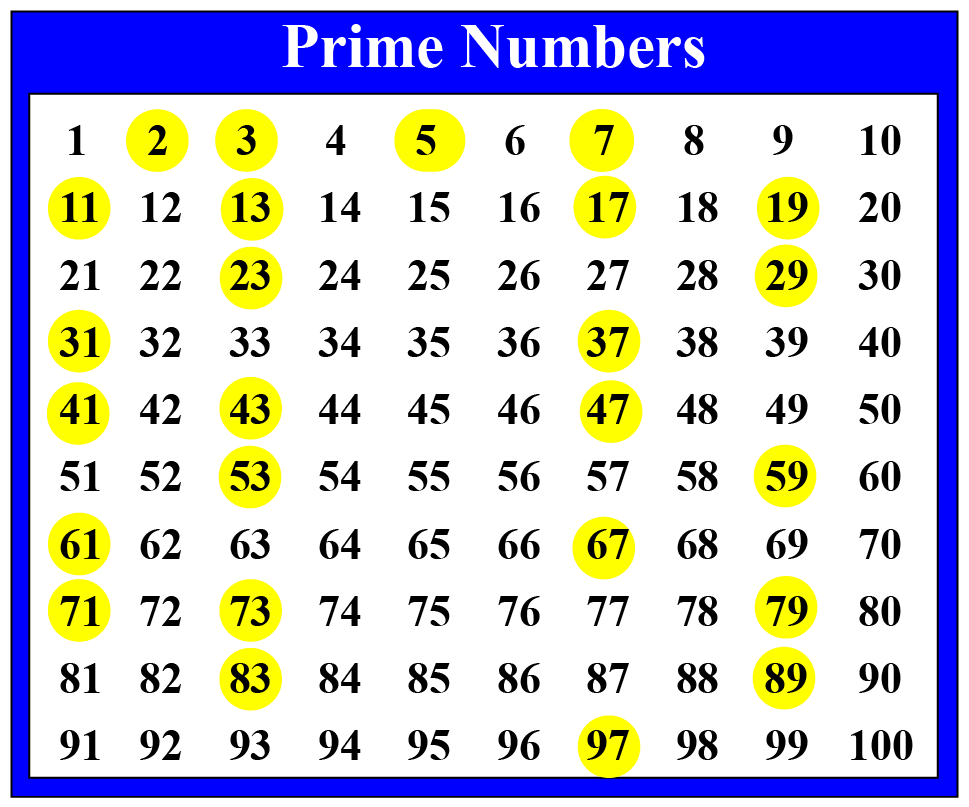# Is 101 a Prime Number ?

Is 101 a Prime Number ?
Go back to  'Prime Numbers'

Rewrite the number 101 from right to left.

Yes, you got the same number right?!Such numbers are called palindrome numbers.

101,1001,1331 are a few examples of palindromes.

101 is a unique palindrome.

Let us explore a little bit more about the number 101

## Lesson Plan

 1 Is 101 a Prime Number? 2 Important Notes 3 Solved Examples 4 Challenging Questions 5 Interactive Questions

## Is 101 a Prime Number?

A prime number is a natural number that has two factors only.

Prime numbers are divisible only by 1 and itself.

Examples: 3,5,7,11 etc;

Let us look at the factors of 101

The factors of 101 are 1,101 i.e., no other factors other than 1 and itself.

So, 101 is a prime number.

## Is 101 a composite number?

101 is not a composite number as it cannot be written as a product of two natural numbers other than 1 and itself.

101 is the smallest 3-digit prime number.

## Solved Examples

 Example 1

kate's mother asked  Kate a question.

"Is 91 a prime number?"

How can Kate decide if 91 is a prime number or a composite number?

Solution

The prime factorization of 91 is 7$$\times$$13

The factors of 91 are 1, 7, 13, 91

So, it is a composite number

 So, 91 is not a prime number
 Example 2

List out the prime numbers between 1 and 100

<< have the chart listing all 1- 100  numbers >>

Solution

Using divisibility rules and the sieve of Eratosthenes we can list the primes below 100.

2, 3, 5, 7, 11, 13, 17, 19, 23, 29, 31, 37, 41, 43, 47, 53, 59, 61, 67, 71, 73, 79, 83, 89, and 97There are 25 primes between 1 and 100Challenging Questions
1. Harry states "Every even number greater than 2 can be written as the sum of two primes". Can you verify his statement?
2. Can a perfect square be the prime number?

## Interactive Questions

Here are a few activities for you to practice.Select/Type your answer and click the "Check Answer" button to see the result.

## Let's Summarize

The mini-lesson targeted the fascinating concept of is 101 a prime number. The math journey around is 101 a prime number starts with what a student already knows, and goes on to creatively crafting a fresh concept in the young minds. Done in a way that not only it is relatable and easy to grasp, but also will stay with them forever. Here lies the magic with Cuemath.

At Cuemath, our team of math experts is dedicated to making learning fun for our favorite readers, the students!

Through an interactive and engaging learning-teaching-learning approach, the teachers explore all angles of a topic.

Be it worksheets, online classes, doubt sessions, or any other form of relation, it’s the logical thinking and smart learning approach that we, at Cuemath, believe in.

## 1. What are co-primes?

Two numbers are said to be co-prime if they do not have any common divisors except for 1

eg: (2,5), (2,9), (7,16) are examples of co-primes.

## 2. Is 101 a prime number and why?

Yes,101 is a prime number as it is not divisible by any number except for 1 and itself.

## 3. Is 101 divisible by any number?

101 is divisible only by 1 and itself.

So, it is a prime number.

More Important Topics
Numbers
Algebra
Geometry
Measurement
Money
Data
Trigonometry
Calculus